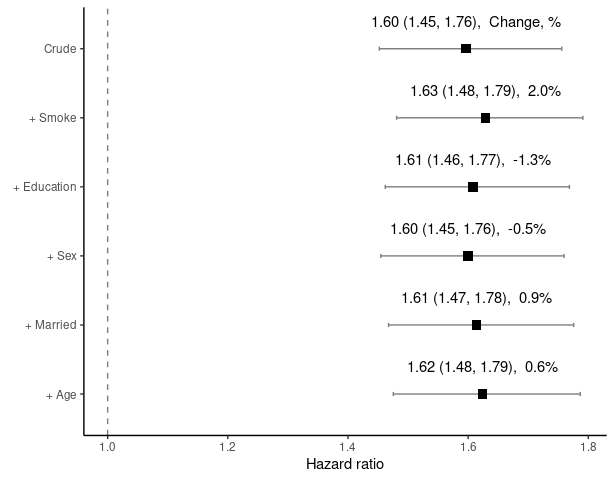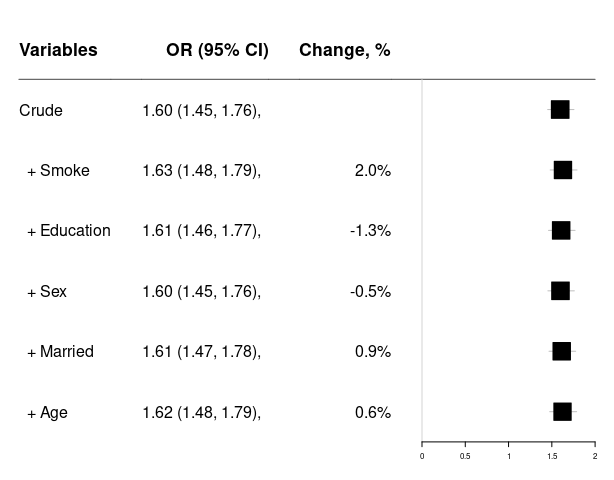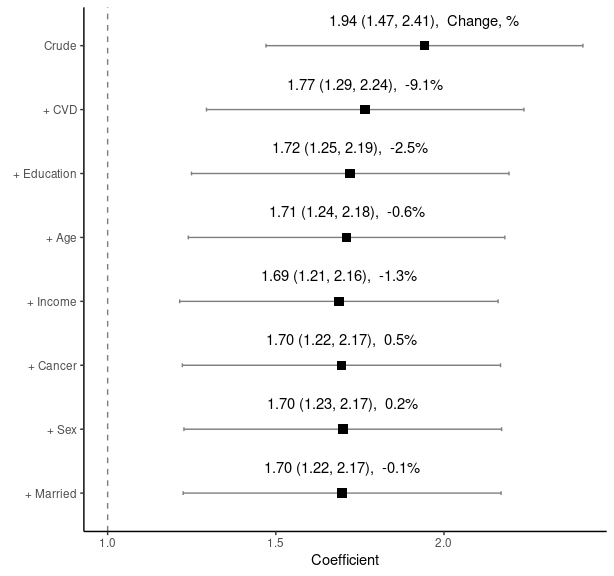# Change-in-estimate Approach: Assessing Confounding Effects

Badges Confounding; Logistic regression; Cox proportional hazards model; Linear regression;

## Overview

The ‘chest’ package systematically calculates and compares effect estimates from various models with different combinations of variables. It calculates the changes in effect estimates when each variable is added to the model sequentially in a step-wise fashion. Effect estimates here can be regression coefficients, odds ratios and hazard ratios depending on modelling methods. At each step, only one variable that causes the largest change among the remaining variables is added to the model. The final results from many models are summarized in one graph and one data frame table. This approach can be used for assessing confounding effects in epidemiological studies and bio-medical research including clinical trials.

## Installation

You can install the released version of chest from CRAN with:

install.packages("chest")

## Getting Started

library(chest)
library(ggplot2)
names(diab_df)
#>   "Endpoint"  "mid"       "Diabetes"  "Age"       "Sex"       "BMI"
#>   "Married"   "Smoke"     "CVD"       "Cancer"    "Education" "Income"
#>  "t0"        "t1"

### Data: diabetes and mortality

A data frame ‘diab_df’ is used to examine the association between Diabetes and mortality Endpoint. The purpose of using this data set is to demonstrate the use of the functions in this package rather than answering any research questions.

### chest_glm: Logistic regression using (generalized linear models, glm).

‘chest_glm’ is slow. We can use indicate = TRUE to monitor the progress.

vlist <- c("Age", "Sex", "Married", "Smoke", "Education")
results <- chest_glm(crude = "Endpoint ~ Diabetes", xlist = vlist,
data = diab_df, indicate = TRUE)

### chest_cox: Using Cox Proportional Hazards Models: ‘coxph’ of ‘survival’ package

vlist <- c("Age", "Sex", "Married", "Smoke", "Education")
results <- chest_cox(crude = "Surv(t0, t1, Endpoint) ~ Diabetes",
xlist = vlist, data = diab_df)
chest_plot(results)chest_forest(results)### chest_clogit: Conditional logistic regression: ‘clogit’ of ‘survival’ package

results <- chest_clogit(crude = "Endpoint ~ Diabetes + strata(mid)",
xlist = vlist, data = diab_df)

### chest_lm: linear regression

vlist<-c("Age", "Sex", "Married", "Cancer", "CVD","Education", "Income")
results <- chest_lm(crude = "BMI ~ Diabetes", xlist = vlist, data = diab_df)
chest_plot(results)